• Data structures play a key role in the programming world. They help us to organize our data in a way that can be used efficiently. The Queue is one of the simplest data structures available.

## What is a Queue?

Queue is a linear data structure that follows the First In/First Out(FIFO) principle. It’s opposite to the Stack data structure.

We can compare the queue with a real-life queue at the cinema ticket counter. Let’s see the illustration of a queue as follows.If you observe the queue at the counter, the second person can go to the counter only after the first person completes his/her work. Here the first person comes to the counter and then the second person. So, here people are following the FIFO(First In/First Out) principle. Whoever comes first, he/she will complete the work first. We can see a similar kind of queues in our day-to-day lives.

The Queue data structure also follows the same principle. Now, you know what queues are and its principle. Let’s see the operations that can be performed on a queue data structure.

## Operations in Queue

Similar to stack, we will find two main operations in a queue data structure.

### enqueue(data)

Adds new data to the queue at the end. The side is called the rear.

### dequeue()

Removes data from the front of the queue. The side is called the front.

Let’s see the queue operations illustrations for better understanding. One picture speaks a thousand words.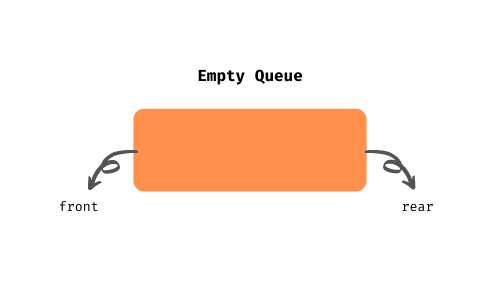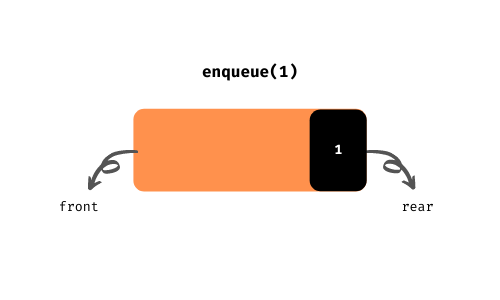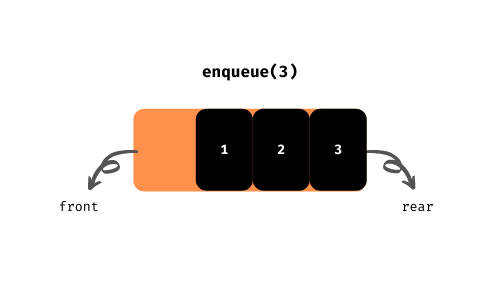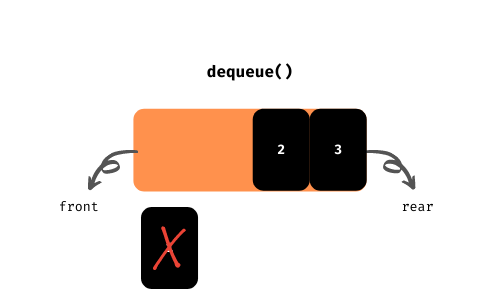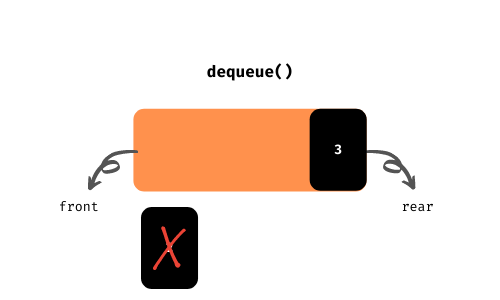We can write some helper functions to get more info about the queue. There is no limit on the number of helper functions you will have. Let’s stick to the most important once mentioned below for now.

### rear()

Returns the first element from the end of the queue.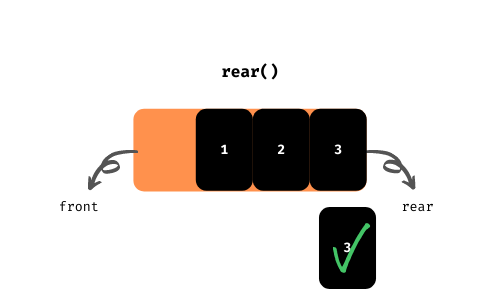### front()

Returns the first element from the start of the queue.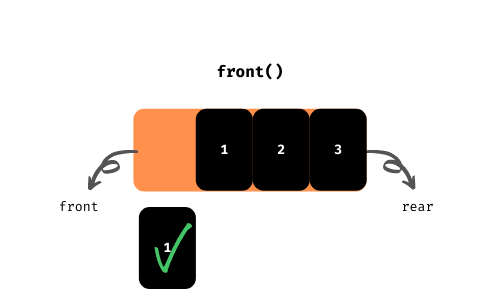### is_empty()

Returns whether the queue is empty or not.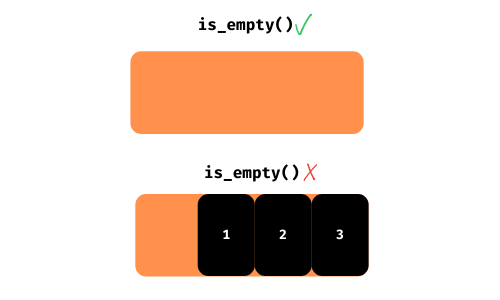Isn’t it boring for you? I mean the conceptual topics.

For me Yes!

But, we can’t do anything without knowing the concepts. We have to complete know it before the implementation. Don’t worry it’s time to make our hands dirty with some code.

I assume you have python installed on your PC if not you can also try the online compiler.

## Queue Implementation

Implementing a queue won’t take more than 15 mins for a programmer. Once you learned, you will say it. Maybe you can implement it within mins after this tutorial.

There are multiple ways to implement the queue in Python. Let’s see different queue implementations step by step.

### #1. List

The list is a built-in data type in Python. We are going to use the list data type to implement a queue in a class. We will walk you through different steps to implement the queue data structure from scratch without any modules. Let’s jump into it.

Step1:

Write a class called Queue.

``````class Queue:
pass``````

Step2:

There has to be some variable to store the data of the queue in the class. Let’s name it elements. And it’s a list of course.

``````class Queue:

def __init__(self):
self.elements = []``````

Step3:

To insert data into the queue, we need a method in the class. The method is called enqueue as we already discussed in the previous section of the tutorial.

The method takes some data and adds it to the queue (elements). We can use the append method of the list data type to add data at the end of the queue.

``````class Queue:

# ...

def enqueue(self, data):
self.elements.append(data)
return data``````

Step4:

The queue needs to have an exit. And that’s called dequeue. I think you already guessed that we are going to use the pop method of the list data type. If you guessed or not, cheers!

The pop method of the list data type deletes an element from the list of the given index. If we didn’t give any index, then it deletes the last element of the list.

Here, we need to delete the first element of the list. So, we have to pass the index to the pop method.

``````class Queue:

# ...

def dequeue(self):
return self.elements.pop(0)
``````

That’s enough for a queue. But, we need the helper functions to test whether the queue operations are working properly or not. Let’s write the helper functions as well.

Step5:

The method rear() is used to get the last element of the queue. Negative indexing in list data type helps us to get the last element of the queue.

``````class Queue:

# ...

def rear(self):
return self.elements[-1]
``````

Step6:

The method front() is used to get the first element of the queue. We can get the first element of the queue using the list index.

``````class Queue:

# ...

def front(self):
return self.elements
``````

Step7:

The method is_empty() is used to check whether the queue is empty or not. We can check for the length of the list.

``````class Queue:

# ...

def is_empty(self):
return len(self.elements) == 0
``````

Phew! completed the implementation part of the queue data structure. We need to create an object for the Queue class to use.

Let’s do it.

``````class Queue:

# ...

if __name__ == '__main__':
queue = Queue()``````

Now, we have a Queue object. Let’s test it with some series of operations.

• Check whether the queue is empty or not using the is_empty method. It should return True.
• Add the numbers 1, 2, 3, 4, 5 to the queue using the enqueue method.
• The is_empty method should return False. Check it.
• Print the front and rear elements using the front and rear methods respectively.
• Remove the element from the queue using the dequeue method.
• Check the front element. It should return the element 2.
• Now, remove all the elements from the queue.
• The is_empty method should return True. Check it.

I think that’s enough to test our queue implementation. Write the code for each step mentioned above to test the queue.

Did you write the code?

No, don’t worry.

Check the code below.

``````class Queue:

def __init__(self):
self.elements = []

def enqueue(self, data):
self.elements.append(data)
return data

def dequeue(self):
return self.elements.pop(0)

def rear(self):
return self.elements[-1]

def front(self):
return self.elements

def is_empty(self):
return len(self.elements) == 0

if __name__ == '__main__':
queue = Queue()

## checking is_empty method -> True
print(queue.is_empty())

queue.enqueue(1)
queue.enqueue(2)
queue.enqueue(3)
queue.enqueue(4)
queue.enqueue(5)

## again checking is_empty method -> False
print(queue.is_empty())

## printing the front and rear elements using front and rear methods respectively -> 1, 5
print(queue.front(), end=' ')
print(queue.rear())

## removing the element -> 1
queue.dequeue()

## checking the front and rear elements using front and rear methods respectively -> 2 5
print(queue.front(), end=' ')
print(queue.rear())

## removing all the elements
queue.dequeue()
queue.dequeue()
queue.dequeue()
queue.dequeue()

## checking the is_empty method for the last time -> True
print(queue.is_empty())``````

I think you run the above program. You can get an output similar to the following result.

``````True
False
1 5
2 5
True``````

We can directly use the list data type as a queue data structure. The above implementation of the queue helps you better understand the queue implementation in other programming languages.

You can also use the above class implementation of a queue in a different program of a project by simply creating the object as we do earlier.

We have implemented the queue from scratch using the list data type. Are there any built-in modules for the queue? Yeah! we have built-in queue implementations. Let’s see them.

### #2. deque from collections

It is implemented as a double-ended queue. Since it supports the addition and removal of elements from both ends, we can use it as a stack and queue. Let’s see the queue implementation using dequeue.

It is implemented using other data structures called the doubly-linked list. So the performance of the insertion and deletion of elements are consistent. Accessing elements from the middle linked list took O(n) time. We can use it as a queue as there is no need to access the middle elements from the queue.

Let’s check the different methods that the dequeue offers us.

• append(data) – used to add the data to the queue
• popleft() – used to remove the first element from the queue

There are no alternative methods for the front, rear, and is_empty. We can print the whole queue in place of front and rear methods. Next, we can use the len method to check whether the queue is empty or not.

We have followed a series of steps to test the queue implementation in the previous. Let’s follow the same series of steps here as well.

``````from collections import deque

## creating deque object
queue = deque()

## checking whether queue is empty or not -> True
print(len(queue) == 0)

## pushing the elements
queue.append(1)
queue.append(2)
queue.append(3)
queue.append(4)
queue.append(5)

## again checking whether queue is empty or not -> False
print(len(queue) == 0)

## printing the queue
print(queue)

## removing the element -> 1
queue.popleft()

## printing the queue
print(queue)

## removing all the elements
queue.popleft()
queue.popleft()
queue.popleft()
queue.popleft()

## checking the whether queue is empty or not for the last time -> True
print(len(queue) == 0)``````

You will get a result similar to the following output.

``````True
False
deque([1, 2, 3, 4, 5])
deque([2, 3, 4, 5])
True``````

### #3. Queue from queue

Python has a built-in module called queue that serves a class called Queue for the queue implementation. It’s similar to the one we have implemented before.

First, let’ checkout different methods of the Queue class.

• put(data) – adds or pushes the data to the queue
• get() – removes the first element from the queue and returns it
• empty() – returns whether the stack is empty or not
• qsize() – returns the length of the queue.

Let’s implement the queue with the above methods.

``````from queue import Queue

## creating Queue object
queue_object = Queue()

## checking whether queue is empty or not -> True
print(queue_object.empty())

queue_object.put(1)
queue_object.put(2)
queue_object.put(3)
queue_object.put(4)
queue_object.put(5)

## again checking whether queue is empty or not -> False
print(queue_object.empty())

## removing all the elements
print(queue_object.get())
print(queue_object.get())
print(queue_object.get())

## checking the queue size
print("Size", queue_object.qsize())

print(queue_object.get())
print(queue_object.get())

## checking the whether queue_object is empty or not for the last time -> True
print(queue_object.empty())
``````

Check the output of the above code.

``````True
False
1
2
3
Size 2
4
5
True``````

There are some other methods in the Queue class. You can explore them using the dir built-in function.

### Conclusion

I hope you have learned about the queue data structure and its implementation. That’s it for the queue. You can use the queue in different places where there need to be processed in FIFO(First In/First Out) order. Use queue in problem-solving whenever you get the case to use it.

Interested in mastering Python? Check out these learning resources.

Happy Coding 🙂 👨‍💻